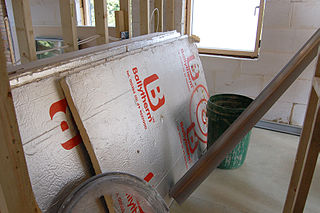# R-value

(diff) ← Older revision | Latest revision (diff) | Newer revision → (diff)Figure 1. Polyisocyanurate insulation, this type of insulation is similar to polyurethane but has a higher R-value.

The R-value of insulation is a value that is used to measure how well a specific type of insulation can resist heat flow. The higher the R-value, the more effective the material is at preventing heat transfer. R-value is short for Resistance value, and is closely related to the metric unit of RSI. Windows also have a specific R-value associated with how well they keep heat in (although the heat that comes from sunlight coming through the window is not included in R-values, it's an important part of the net effect of having a window in a particular wall). R-value is also related to U-value (which is 1/R-value) and thermal conductivity which is a property of a material that says how well it conducts heat.

Different insulating materials each have their own unique R-value per unit length. The thicker the material the more it resists heat transfer so values are listed per inch (and then multiplying the value by the thickness of the insulation gives the R-value). Some of the best insulation materials for buildings are:

• Blown in Cellulose Insulation: 3.70/inch
• Fiberglass Insulation: 3.14/inch
• Expanded Polystyrene: 4.00/inch

R-values, as shown above, are expressed in imperial units, specifically °F ft2 hr/BTU. R-values do however have a metric equivalent, known as the RSI value (which are °C m2 sec/J). Insulating products sold in Canada are labelled with both R and RSI values for clarity.

In Canada, provincial building codes specify minimum R/RSI values for new construction. The recommended R-value for basement insulation in North America is R12, but the R-value in insulation is lowered substantially when there is air or moisture leaks. Thus the standard R-value varies by climate, temperature, and specific conditions.

Although effective, looking at R-value alone isn't enough to determine how well an insulation will work. Since heat moves by conduction, convection, radiation, and air infiltration, all of these must be accounted for to truly know how well any one insulation will work. R-value measures only conduction, and thus doesn't provide the whole picture. As well, R-value doesn't take into account individual factors such as wind, humidity, and temperature changes which can cause air flow and leakages in the insulation of the building. These factors change how well a specific type of insulation works, effectively changing it's R-Value, but this is very hard to measure.

## Calculating R-value

Mathematically, R-value is calculating from a measuring heat flow across a surface where each side is at a particular temperature:

• is the temperature difference inside and outside the house in °F (or °C for RSI)
• is the area of the wall (or ceiling) that's being insulated in ft 2 (or m2 for RSI)
• is how long the measurement took place in seconds (same in RSI)
• is how much heat is lost through the wall in BTU (J in RSI)

To convert from an RSI value to an R-value multiply by 6. If one insulation material is put on top of another insulation material, the R-values just add. For a helpful online R-value calculator from Ekotrope, click here.